# 2 - Running Segmentation/Clustering with segclust2d

#### 2023-08-21

library(segclust2d)

The package segclust2d provides access to two algorithms:

• It can perform a segmentation of the time-series into homogeneous segments. A typical case is the identification of home-range shifts

• It can also perform an integrated classification of those segments into clusters of homogeneous behaviour through a segmentation/clustering algorithm. This can be used to identify behavioural modes.

Input data used in the examples here is a data.frame object but it can also be a Move object, a ltraj object (from package adehabitatLT) or a sftraj object, both shown in section Preparing data for Segmentation/Clustering with segclust2d

# Presentation of data sets

## simulshift: three successive home-range shifts

data(simulshift)

Here we will load a test dataset: simulshift, containing a simulation of home-range behaviour with two shifts. It is a data.frame with two columns for coordinates : x and y. We can now run a simple segmentation with this dataset to find the different home-ranges.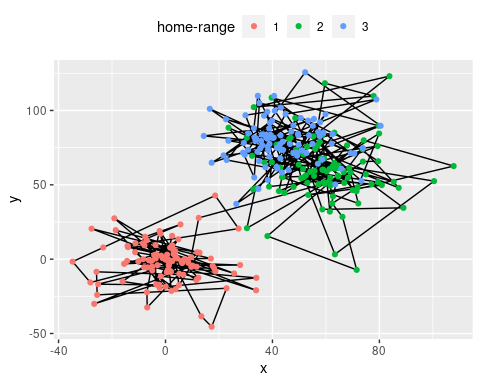simulshift: simulation of movement within three successive home-range. Data shown after subsampling by 100.

## simulmode: a simulation with three movement modes

data(simulmode)
simulmode$abs_spatial_angle <- abs(simulmode$spatial_angle)
simulmode <- simulmode[!is.na(simulmode\$abs_spatial_angle), ]

simulmode is an example dataset containing a movement simulation with three movement modes. It is a data.frame with 11 columns, with location coordinates and several covariates. In addition to loading we calculated here the absolute value for the turning angle at constant step length (here called spatial_angle). Data are also checked for missing values.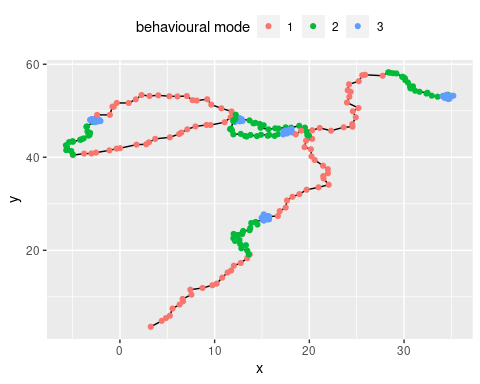simulmode: simulation of movement with three different behavioural modes.

# Segmentation

## Setting segmentation() parameters.

### Required: lmin: minimum length of a segment

To run the segmentation() function, argument lmin needs to be provided. lmin is the minimum length of a segment. It is has to be set not only to speed up the algorithm, but also, more fundamentally, to prevent over‐segmenting, based on biological considerations. For example, setting lmin to a value of a few weeks when analysing locational time series will prevent the algorithm from considering an area exploited only for a few days, corresponding to foray outside the usual home range or to stopover during migration, as a distinct home range. Similarly, setting lmin to a value long enough (depending on the species) when looking for changes of behavioural modes will force the algorithm to assign a given behavioural bout to a given mode even when it is interspersed by ephemeral events related to another behaviour (e.g. a long transit with opportunistic short feeding events on the move will be considered as a single transit phase).

Statistically, lmin cannot be <5, because of the need to estimate variances for each segment. To avoid such case, the program will fix lmin to a minimum of 5.

### Optional: Kmax: the maximum number of segments.

By default, Kmax will be set to 0.75*floor(n/lmin), with n the number of observations, so you can omit this argument. You can however provide a different value, as a large value may compromise speed or selection of optimal number of segments.

### Optional: seg.var

You can specify the variables to be segmented using argument seg.var. By default, function segmentation will use variables x and y for a data.frame or use the coordinates for move and ltraj objects.

### Optional: scale.variable

The function allow rescaling of variable with argument scale.variable. Rescaling sets variable in seg.var to a mean of 0 and a variance of 1. It is recommended if the variables chosen for segmentation are of different nature (e.g. speed and turning angle). It is therefore inadvisable for segmentation of coordinates to identify home-ranges. By default segmentation() disables rescaling and sets scale.variable = FALSE.

### Optional: diag.var

segmentation() uses variables provided in seg.var and automatically produces summary statistics for each variable and each segments (mean and standard deviation). In case you want summary statistics for other variables than seg.var you can specify them in diag.var. By default diag.var is set to seg.var.

### Optional: order.var

order.var is the variable used for ordering segments in some of the output functions (see section Exploring Outputs from segclust2d for details.). By default order.var is set to seg.var

### Deprecated: type, coord.names

Older version of segclust2d had different arguments, now deprecated. type was either "home-range" or "behaviour", which provided different default arguments:

• type = "home-range" was associated with scale.variable = FALSE and performed a segmentation based on the variables proposed as coord.names. Default value was coord.names = c("x","y").
• type = "behaviour" was associated with, scale.variable = TRUE and performed a segmentation based on the variables proposed as seg.var, with no default value.

From version 0.3.0 and forward, those arguments were removed so that the functions now work only with arguments scale.variable and seg.var. Using the arguments type makes the function fail and triggers message explaining the required changes:

df.seg <- segmentation(simulshift,
type = "home-range",
lmin = 300, Kmax = 10,
subsample_by = 60)
#> ✖ Argument type is deprecated and should not be used
#> ✖ Argument coord.names is deprecated and should not be used
#> → Please use instead seg.var = c("x", "y") and scale.variable = FALSE

## Running segmentation()

segmentation() uses a Dynamic Programming algorithm that finds the best segmentation given a number of segments. The function runs the dynamic programming for each number of segments <Kmax. As a result, the optimal segmentation is associated with a likelihood value for each number of segment. Section Different running configurations shows several examples of running segmentation() with different options. Section Selecting the number of segments discusses the selection of the number of segments using either Lavielle’s criterium or graphical explorations.

### Different running configurations

In this section we will try different ways of calling the segmentation() function. Minimal call only contains the data concerned (here the data.frame simulshift) and argument lmin. The function will automatically complete the missing arguments with default values and communicate about it (not shown here).

subsample_by argument is described in section Subsampling

shift_seg <- segmentation(simulshift,
lmin = 240,
subsample_by = 60)

It is however advised to give a more reasonable ‘Kmax’ value to decrease calculation time.

shift_seg <- segmentation(simulshift,
lmin = 240, Kmax = 25,
subsample_by = 60)

There is a check to ensure that lmin*Kmax < n with n the number of data. If you provided inadequate values, Kmax will be adjusted to an appropriate value if possible and you should get a message like this:

#> ! Adjusting Kmax so that lmin*Kmax < nrow(x). Now, Kmax = 25

If not possible you will get an error and this message:

#> ✖ lmin*Kmax > nrow(x) and Kmax cannot be adjusted. Please provide lower values for lmin
#> Error in eval(expr, envir, enclos): lmin*Kmax > nrow(x)

By default the function is looking for column c(x,y) or the coordinates (in the case of a Move or a ltraj object). Alternatively the user can provide its own variables, depending on its aim:

shift_seg <- segmentation(simulshift,
seg.var = c("x","y"),
lmin = 240, Kmax = 25,
subsample_by = 60)

### Selecting the number of segments

Once the segmentation have been successfully run, a summary like this will appear

#> ✔ Best segmentation estimated with 3 segments, according to Lavielle's criterium
#> Other number of segments may be selected by looking for likelihood breaks with
#> plot_likelihood()
#> Results of the segmentation may be explored with plot() and segmap()

By default, the algorithm chooses the number of segments given a criterium developed by Marc Lavielle based on the value of the second derivative of the penalized likelihood. This criterium uses a threshold value of S = 0.75, but a different threshold can be specified to segmentation() if needed.

As stated it is important to check that the number of segments selected corresponds to a clear break in log-likelihood and if it is not the case to select a better value. This can be checked with plot_likelihood that shows the log-likelihood of the best segmentation versus the number of segments and highlights the one chosen with Lavielle’s criterium. The likelihood should show an increasing curve with a clear breakpoint for the optimal number of segments. Note that with real data breaks are often less clear than for that example. An artifactual decrease of likelihood can happen for large number of segments when Kmax is too high (close to n/lmin) and corresponds generally to an oversegmentation (in such case, Kmax should be decreased).

plot_likelihood(shift_seg)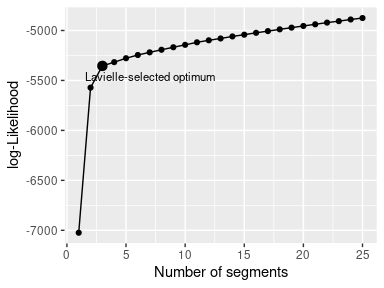Another example on the simulmode dataset shows a situation where the number of segments automatically selected by Lavielle’s criterium is 1, despite a (relatively) clear break in the likelihood at nseg = 15. In such case it is of paramount importance to explore the log-likelihood to confirm or select an appropriate number of segments.

Note that in this case the problem in the selection of the number of segments would have been avoided by setting Kmax = 25. For Lavielle’s criterium to work properly Kmax should be clearly larger than your expected number of segment.


mode_seg <- segmentation(simulmode,
lmin = 10, Kmax = 20,
seg.var = c("speed","abs_spatial_angle"),
scale.variable = TRUE)

plot_likelihood(mode_seg)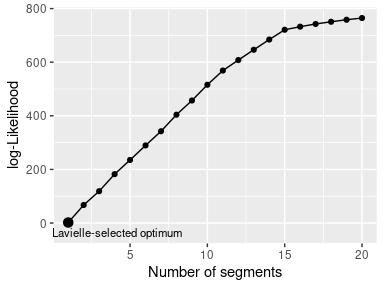# Segmentation-Clustering

We will now run the joint segmentation/clustering segclust() function on the simulmode data to identify the different behavioural modes. As with segmentation(), you can specify the variables to be segmented using argument seg.var.

## Setting segclust() parameters.

segclust() shares most of its parameters with segmentation() so you can read section Setting segmentation() parameters. for the parameters missing description here. The only additional parameter is the number of clusters to be tested.

### Required: ncluster: the number of clusters

ncluster is an argument required for segclust(). The user can provide a vector of values or a single value. The algorithm provides a BIC-criterium to select the number of clusters, although it is advised to select it based on biological knowledge.

## Running segclust()

### Different running configurations

In this section we will try different ways of calling the segclust() function. Minimal call only contains the data concerned (here the dataframe simulmode) as well as arguments lmin, ncluster and seg.var. The function will automatically complete the missing arguments with default values and communicate about it (not shown here).

mode_segclust <- segclust(simulmode,
Kmax = 20, lmin=10,
ncluster = c(2,3),
seg.var = c("speed","abs_spatial_angle"))

Additionally it is advised to scale the variables used to a mean of 0 and a variance of 1. This will automatically be done for segclust(), but can also be specified explicitly:

mode_segclust <- segclust(simulmode,
Kmax = 20, lmin=10,
ncluster = c(2,3),
seg.var = c("speed","abs_spatial_angle"),
scale.variable = TRUE)

### Selecting the number of clusters and segments.

Once the segmentation/clustering have been successfully run. A summary like this will appear:

#> ✔ Best segmentation/clustering estimated with 3 clusters and 15 segments according to BIC
#> → Number of clusters should preferentially be selected according to biological
#> knowledge. Exploring the BIC plot with plot_BIC() can also provide advice to
#> select the number of clusters.
#> → Once number of clusters is selected, the number of segments can be selected
#> according to BIC.
#> → Results of the segmentation/clustering may further be explored with plot()
#> and segmap()

As stated, the number of clusters should preferentially be selected based on biological knowledge. Exploration of the BIC-based penalized log-likelihood can also help selecting an appropriate number of clusters, with the function plot_BIC(). Best-case scenario is as below, the BIC shows a steep increase up to a maximum and a slow decrease after the optimum and one number of clusters is clearly above the others.

plot_BIC(mode_segclust)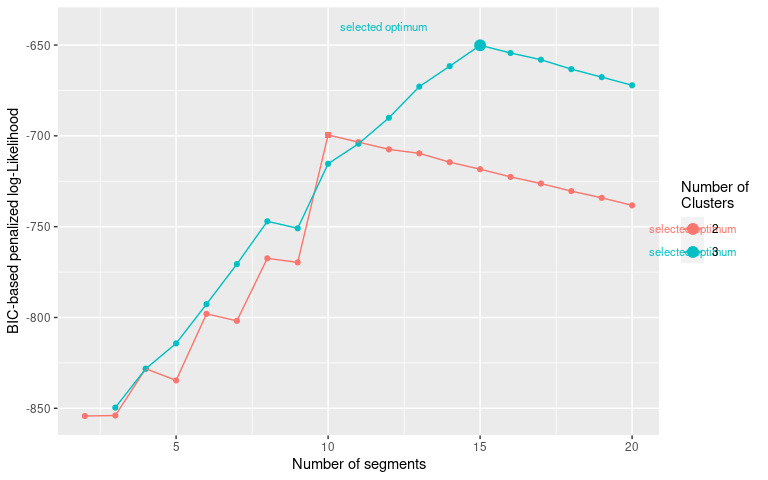With real data, a larger number of clusters almost always improves the penalized-likelihood so it will generally be a poor indication of the appropriate number of clusters. Such situation may be shown with our dataset simulmode indeed, if we allow the number of clusters to be tested between 2 and 5 instead of a maximum at three, we obtain the following BIC curve:

mode_segclust <- segclust(simulmode,
Kmax = 20, lmin=10,
ncluster = 2:5,
seg.var = c("speed","abs_spatial_angle"),
scale.variable = TRUE)

plot_BIC(mode_segclust)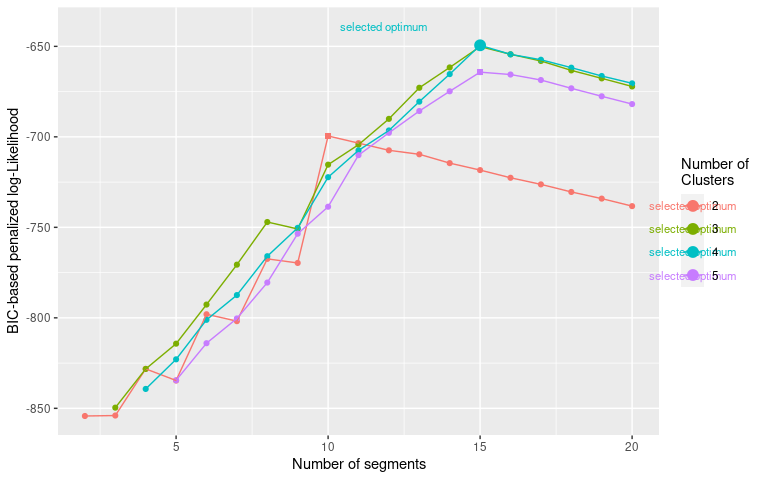In the curve above we see that the optimum number of clusters selected is 4, despite the difference being relatively small compared to ncluster = 3 and to the evolution with the number of segments. In addition, if we look at the results of the segmentation we can see identical segment distribution, the only difference is that state 2 in the segmentation with 3 clusters is divided into state 2 and 3 in the segmentation with 4 clusters:

plot(mode_segclust, ncluster = 3)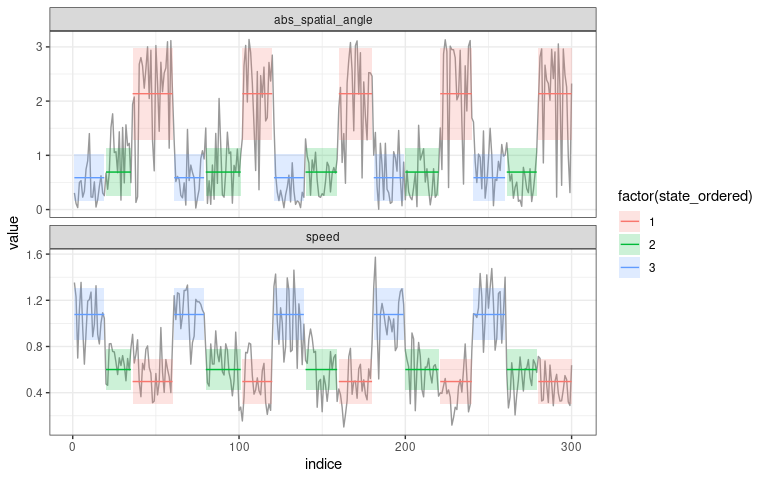plot(mode_segclust, ncluster = 4)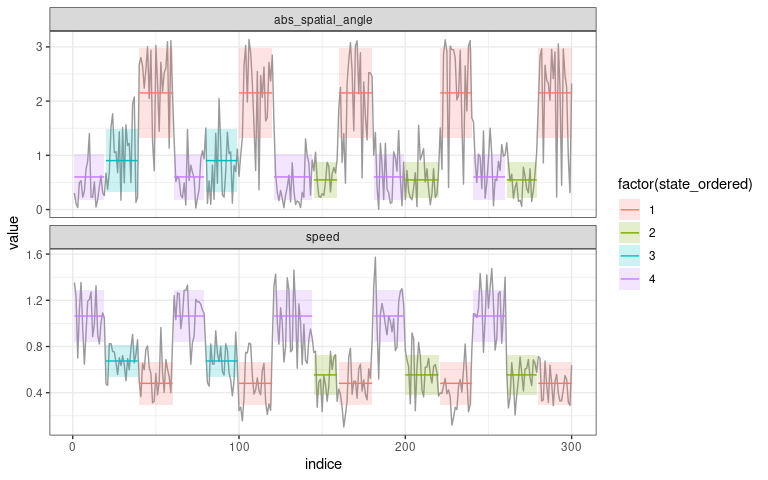More generally, as in this example, if the selected number of segments for a higher number of clusters is the same, then the lower number of clusters should be favored.

Once the number of clusters have been selected, the number of segments can be selected with the BIC criterium. When the segmentation-clustering is reliable, the selected optimum should be a maximum just before a linear drop of the penalized log-Likelihood as in the examples above. With real data the signal is seldom as clear and may require adjustment based on exploration of the realized segmentation/clustering.